# Algebra II : Graphing Circular Inequalities

## Example Questions

← Previous 1

### Example Question #1 : Graphing Circular Inequalities

Find an inequality for points on a graph that fall on or inside of a circle centered at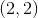with a radius of, as shown below.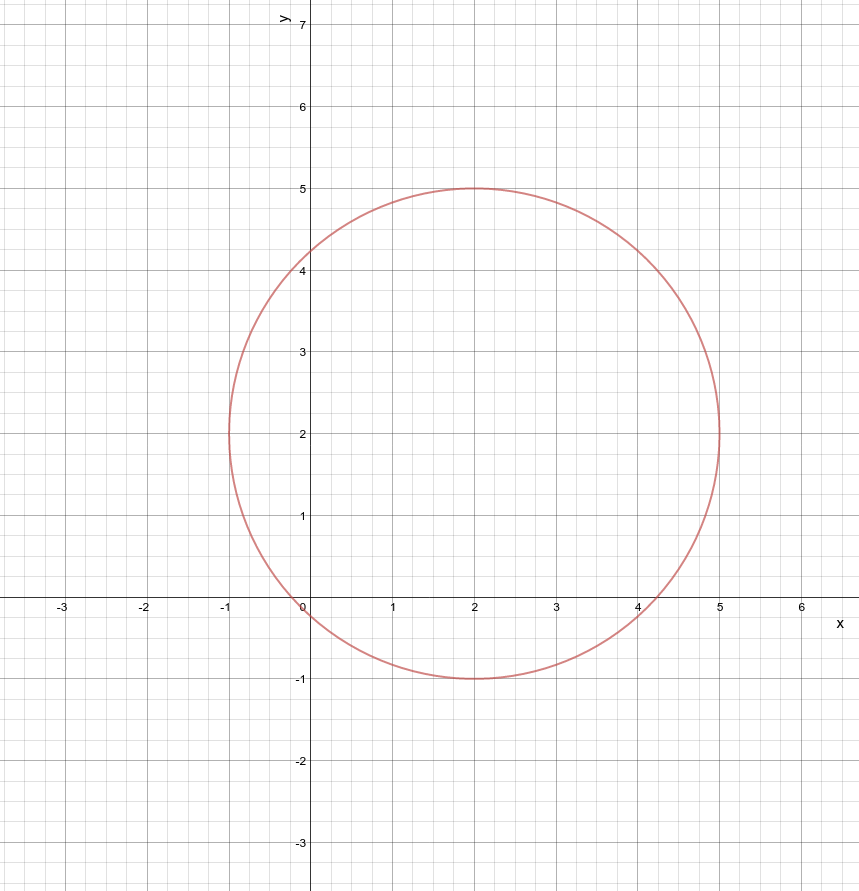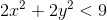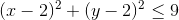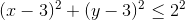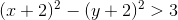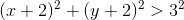Explanation:

The equation for a circle centered at point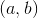with radiusis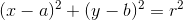. Our circle is centered atwith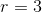, and we are interested in points that lie along or inside of the circle. This means the left-hand side must be less than or equal to the right-hand side of the equation. We are left with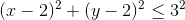or### Example Question #2 : Graphing Circular Inequalities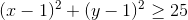Given the above circle inequality, which point is not on the edge of the circle?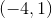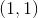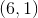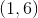Explanation:

This is a graph of a circle with radius of 5 and a center of (1,1). The center of the circle is not on the edge of the circle, so that is the correct answer. All other points are exactly 5 units away from the circle's center, making them a part of the circle.

### Example Question #1 : Graphing Circular InequalitiesGiven the above circle inequality, does the center satisfy the equation?

Maybe

Yes

Can't tell

No

No

Explanation:

The center of the circle is, so plugging those values in for x and y yields the response that 0 is greater than or equal to 25.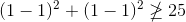Since plugging in the center values gives us a false statement we know that our center does not satisfy the inequality.

### Example Question #11 : Quadratic InequalitiesGiven the above circle inequality, is the shading on the graph inside or outside the circle?

Both

Outside

Can't Tell

Inside

Outside

Explanation:

Check the center of the circle to see if that point satisfies the inequality.

When evaluating the function at the center (1,1), we see that it does not satisfy the equation, so it cannot be in the shaded region of the graph.

Therefore the shading is outside of the circle.

### Example Question #5 : Graphing Circular Inequalities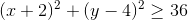Given the above circle inequality, which point is not on the edge of the circle?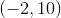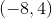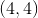Explanation:

This is a graph of a circle with radius of 6 and a center of (-2,4). The point (2,2) is not on the edge of the circle, so that is the correct answer. All other points are exactly 6 units away from the circle's center, making them a part of the circle.

### Example Question #1051 : Algebra IiGiven the above circle inequality, which point satisfies the inequality?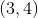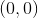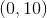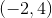Explanation:

The left side of the equation must be greater than or equal to 36 in order to satisfy the equation, so plugging in each of the values for x and y, we see: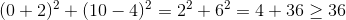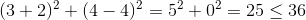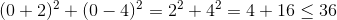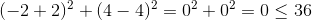Therefore onlyyields an answer that is greater than or equal to 36.

### Example Question #1 : Graphing Circular InequalitiesGiven the above circle inequality, does the center satisfy the equation?

Can't tell

Yes

Maybe

No

No

Explanation:The center of the circle is, so plugging those values in for x and y yields the response,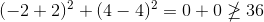Therefore, the center does not satisfy the inequality.

### Example Question #1 : Graphing Circular InequalitiesGiven the above circle inequality, is the shading on the graph inside or outside the circle?

Can't tell

Outside

Inside

Both

Outside

Explanation:

Check the center of the circle to see if that point satisfies the inequality. When evaluating the function at the center (-2,4), we see that it does not satisfy the equation, so it cannot be in the shaded region of the graph. Therefore the shading is outside of the circle.

### Example Question #9 : Graphing Circular Inequalities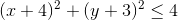Given the above circle inequality, which point is not on the edge of the circle?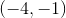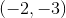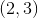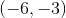Explanation:

Recall the equation of a circle: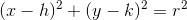where r is the radius and (h,k) is the center of the circle.

This is a graph of a circle with radius of 2 and a center of (-4,-3). The point (2,3) is not on the edge of the circle, so that is the correct answer.

All other points are exactly 2 units away from the circle's center, making them a part of the circle's edge.

### Example Question #1 : Graphing Circular InequalitiesGiven the above circle inequality, which point satisfies the inequality?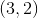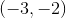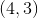Explanation:

The left side of the equation must be less than or equal to 4 in order to satisfy the equation, so plugging in each of the values for x and y, we see: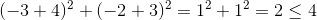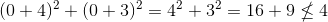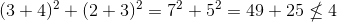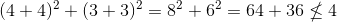The only point that satisfies the inequality is the point (-3,-2), since it yields an answer that is less than or equal to 4.

← Previous 1

### All Algebra II Resources